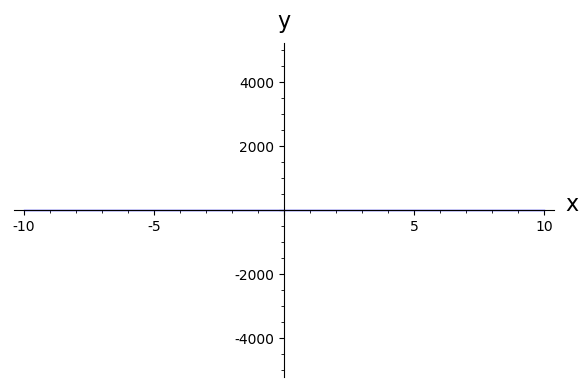CoCalc Public FilesTA sandbox / Hao's sandbox / Slides / Lab02_slides / Lab02_slides_v2.ipynb
Author: HAO LEE
Views : 81

# Review

### Continuous Time System

• What is the difference between R' and R?
• What is a, b, c, d, e, f?

Ans:

• R is a state variable, R' is the rate of change
• a, e can be per-captia rate or proportionality, b and d are mostly proportionality, and c and f can be constant growth rate.

## Nomenclature

• Per-captia rate: increase/ decrease rate per unit of itself.
• Proportionality: increase/ decrease rate related to anything.

## e.g.,

• R's growth rate is proportional to its own population, with proportionality a
• R's per-captia growth rate is a
• R's growth rate is proportional to J, with proportionality b
• R has a constant growth rate c

# Programming

## Type of Data

• Variables have data types
var1 = 1
var2 = 'some string'
print(var1 + var2)


## Data Types of Variables

In :
a = 1
print(type(a))
b = 'I am a string'
print(type(b))

<type 'sage.rings.integer.Integer'> <type 'str'>

## List

#### Put multiple variables together

In :
var1 = 1
var2 = 2
var3 = 3
my_array = [var1, var2, var3]
show(my_array)

$\left[1, 2, 3\right]$

# It does not need to be the same data type

In :
var1 = 1
var2 = 'String'
var3 = plot(x^2,(x,-2,2))

my_list= [var1,var2,var3]
print(my_list)

[1, 'String', Graphics object consisting of 1 graphics primitive]

# How can we retrieve variables from a list?

#### => Indexing

In :
print(my_list)
show(my_list)


[1, 'String', Graphics object consisting of 1 graphics primitive]# How Indexing Works?• If starts from the beginning, index starts from 0
• If starts from the end, index starts from -1

## Example

In :
my_list = [1,2,3,4,5]
print(my_list)
print(my_list)
print(my_list[-2])

[1, 2, 3, 4, 5] 1 4

## We can retrieve more than one element!

In :
my_list[1:4]

[2, 3, 4]

Be careful, for index range 1:4, only elements at position 1,2,3 are retrieved.

## Add components into a list

In :
print(my_list)
my_list.append(9)
print(my_list)

[1, 2, 3, 4, 5] [1, 2, 3, 4, 5, 9]

## Plot of List

### Currently we have learned plot

plot(function,(x,x_min,x_max),options)


##### For most data set, we do not have the function!

Example: For a data set (x1,x2,x3,x4.......,xn),(y1,y2,y3,y4,........yn), please find their relationship!

X= (1,2,3,4,5)

Y= (1,4,9,16,25)

In :
listToPlot=[(1,1),(2,4),(3,9),(4,16),(5,25)]
fig = list_plot(listToPlot)
show(fig)## (,) IS ANNONYING

In :
X = (1,2,3,4,5)
Y=(1,4,9,16,25)
listToPlot=zip(X,Y)
listToPlot  #just wrote the variable's name can also print it!
list_plot(listToPlot,axes_labels=['Index','Value'],title='Test Data')
# take a look at the new options we set!!!!!## Connect all the points

In :
X = (1,2,3,4,5)
Y=(1,4,9,16,25)
listToPlot=zip(X,Y)
list_plot(listToPlot,axes_labels=['Index','Value'],title='Test Data',plotjoined=True)## Loops

### Whatis loops for?

=> Doing Repeated Task for Different Things

for var in list:
do something to var

In :

listVar=[1,2,3,4,5,6]

curSum = 0
for var in listVar:
curSum = curSum+var
print(curSum)

1 3 6 10 15 21

## Create list in a Loop

This is very useful when we are trying to do the following operation

for testObj in list_of_testObj:


We want to record the results

## e.g., Do square root to [1,2,3,4,5,6,7]

In :
allResult=[] #this is call an empty list
in_list = [1.,2.,3.,4.,5.,6.,7.]
for element in in_list:
allResult.append(sqrt(element))
print(allResult)

[1.00000000000000, 1.41421356237310, 1.73205080756888, 2.00000000000000, 2.23606797749979, 2.44948974278318, 2.64575131106459]

## Animation

### What animation does?

Create a list of plots and plot them!!!!!

In :
plots=[]
power =range(15)
for z in power:
p=plot(x^z,(x,-10,10),axes_labels=['x','y'],ymax=5000,ymin=-5000)
plots.append(p)
a=animate(plots)
show(a)In :
listVar = [1,2,3]
for var in listVar:
print(var)

1 2 3
In [ ]: